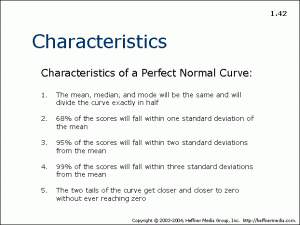# 42: Characteristics of a Perfect Normal Curve## Characteristics

Characteristics of a Perfect Normal Curve:

1. The mean, median, and mode will be the same and will divide the curve exactly in half
2. 68% of the scores will fall within one standard deviation of the mean
3. 95% of the scores will fall within two standard deviations from the mean
4. 99% of the scores will fall within three standard deviations from the mean
5. The two tails of the curve get closer to zero without ever reaching zero